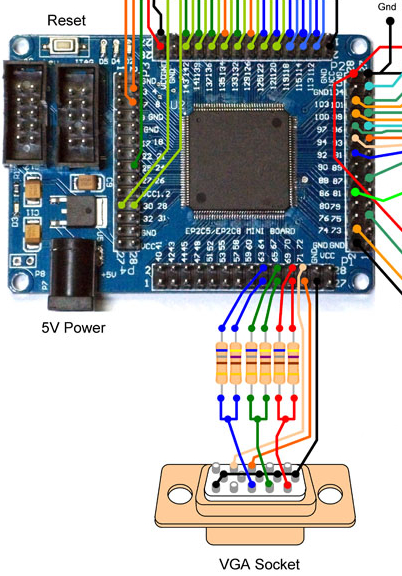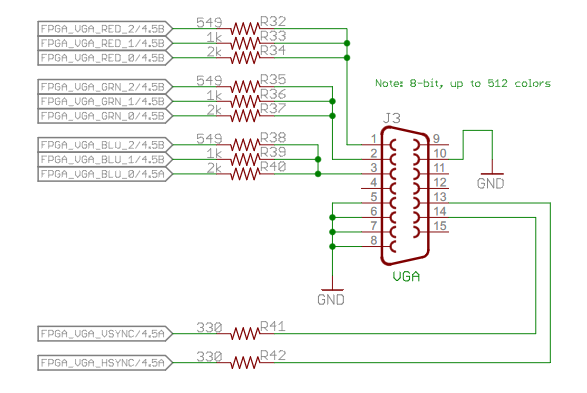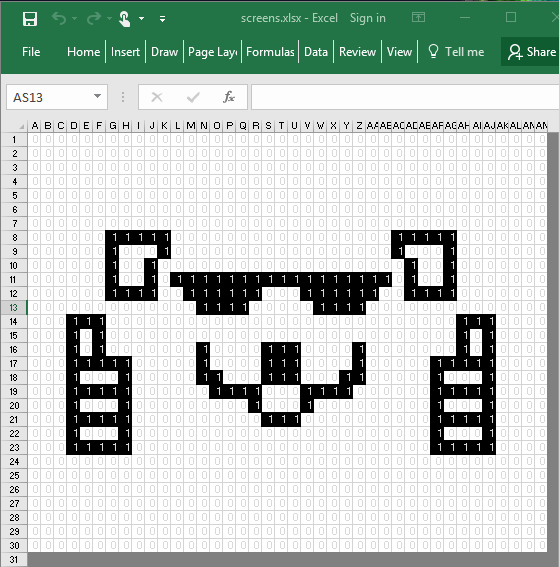# Learning VHDL - VGA

Next in line in the awesome tutorial series from Nandland was VGA. I didn’t have a VGA connector for my FPGA, but wondered if I could jury-rig something with what I had on hand.

The wiring diagram below from Grant Seale’s Multicomp gave me a very good idea on how to go about it.In the Nandland tutorial (image below), three signals are used per channel instead of two so my setup reflects that.I had five 1Kohm resistors on hand, enough to control one channel. I used two of them in parallel to get the 500 ohms (close enough to 549), two in series to get the 2K ohms, and one on its own for the 1K ohms. Jumper wires were used to connect to the pins (red, green, blue, hsync, vsync). Luckily, all the ground pins were in-line (and the random pin in-between was an unused pin) so I just tied the whole line to ground using one wire (instead of several jumper wires). Here is the final masterpiece:

And the overall setup:

In theory it should have worked, but I was very skeptical. Imagine my shock when I uploaded the program to the FPGA, turned on the screen, and saw this:

You know what, I declare the monochrome to be a “feature”! You get your choice (on runtime no less) of three whole colors (ravishing red, gregarious green, and brilliant blue)!

Here’s a video of the test pattern running as shown in the tutorial. There are recording artefacts - the colors while solid in real life, appear distorted in the video.

Pong

With the test patterns working, I moved on to the Pong tutorial.

I modified the code in the tutorial for single player gameplay, and added end screens and score keeping.

_End Screens_

I created the end screens using Excel. It was the simplest thing I could think of to get the zeros and ones (via copy and paste). The dimensions are based on the constants `c_gameWidth` and `c_gameHeight`. Here’s an example of one of the screens:And a portion of the associated code:

``````
architecture arch of DRAW_WIN_SCREEN is

signal colIndex : integer range 0 to 2 ** COL_COUNT_DIV'length := 0;
signal rowIndex : integer range 0 to 2 ** ROW_COUNT_DIV'length := 0;

type pixels is array( 0 to c_gameHeight - 1 ) of std_logic_vector( 0 to c_gameWidth - 1 );
signal pixel : pixels;

begin

-- Screen setup
pixel(0)  <= "0000000000000000000000000000000000000000";
pixel(1)  <= "0000000000000000000000000000000000000000";
pixel(2)  <= "0000000000000000000000000000000000000000";
pixel(3)  <= "0000000000000000000000000000000000000000";
pixel(4)  <= "0000000000000000000000000000000000000000";
pixel(5)  <= "0000000000000000000000000000000000000000";
pixel(6)  <= "0000000000000000000000000000000000000000";
pixel(7)  <= "0000001111100000000000000000111110000000";
pixel(8)  <= "0000001000100000000000000000100010000000";
pixel(9)  <= "0000001001000000000000000000010010000000";
pixel(10) <= "0000001001011111111111111111010010000000";
pixel(11) <= "0000001111001111110001111110011110000000";
pixel(12) <= "0000000000000111100000111100000000000000";
pixel(13) <= "0001110000000000000000000000000001110000";
pixel(14) <= "0001010000000000000000000000000001010000";
pixel(15) <= "0001010000000100001110000100000001010000";
pixel(16) <= "0001111100000100001110000100000111110000";
pixel(17) <= "0001000100000110001110001100000100010000";
pixel(18) <= "0001111100000011110001111000000111110000";
pixel(19) <= "0001000100000000010001000000000100010000";
pixel(20) <= "0001111100000000001110000000000111110000";
pixel(21) <= "0001000100000000000000000000000100010000";
pixel(22) <= "0001111100000000000000000000000111110000";
pixel(23) <= "0000000000000000000000000000000000000000";
pixel(24) <= "0000000000000000000000000000000000000000";
pixel(25) <= "0000000000000000000000000000000000000000";
pixel(26) <= "0000000000000000000000000000000000000000";
pixel(27) <= "0000000000000000000000000000000000000000";
pixel(28) <= "0000000000000000000000000000000000000000";
pixel(29) <= "0000000000000000000000000000000000000000";

colIndex <= to_integer( unsigned( COL_COUNT_DIV ) );
rowIndex <= to_integer( unsigned( ROW_COUNT_DIV ) );

-- Draw screen
process( CLK )

begin

if rising_edge( CLK ) then

if PLAYER_WON = '1' and pixel( rowIndex )( colIndex ) = '1' then

DRAW_WIN <= '1';

else

DRAW_WIN <= '0';

end if;

end if;

end process;

end architecture;
``````

A similar approach can be used to create text and other graphics.

_Score Keeping_

The code as is in the tutorial doesn’t actually track score properly…

I had set the game to end either when the player misses a ball, or when the player reaches the target score (as set by constant `c_scoreLimit`). However, the game seemed to always end after one successful rebound of the ball regardless of whatever high number I set `c_scoreLimit` to. I tried to logic my way through the code to find out what could be going wrong but found nothing. I then decided to use the onboard LEDs to show the player’s score. This revealed something interesting. The score was always at max when the game ended even though the ball itself had only bounced once against the opposite wall (one successful rebound).

Thinking about it some more, I realized the problem. The ball’s motion was delayed by a counter. The main game code was updated every clock cycle, which in this case was 25 million times per second. If the ball’s position was also updated at this speed, it would be moving too fast to be seen. So a counter is used to reduce the update frequency of the ball, in this case to 20 times a second (the counter counts to 1.25 million before updating the ball’s position). This means that in the time it takes the ball to hit the wall (old position) and then bounce of the wall to a new position, the main game code has been polling the ball’s position 1.25 million times. That is, instead of incrementing the player’s score only once, it increments the player’s score 1.25 million times!

Once I realized this was the problem, the solution was straightforward. I added an output signal to the `BALL_CONTROL` entity. The signal is true only when the ball position is updated, and false otherwise (i.e. when the counter is counting). This signal is then used by the main code to determine when to check for a successful rebound or a miss.

_In action_

Here ares some videos of the game in action. There are recording artefacts - in real life you don’t see the “ghosting” effect on the ball, and instead see one solid square.

Code

Here are links to the code:

Final Thoughts

I’ve reached the end of the Nandland tutorials, so next up hopefully is the FPGA controlling some key elements of the homebrew computer.

Nandland turned out to be an amazing resource for learning the VHDL and Verilog hardware description languages. I highly recommend it. Apart from the tutorial series, the site has numerous helpful articles.